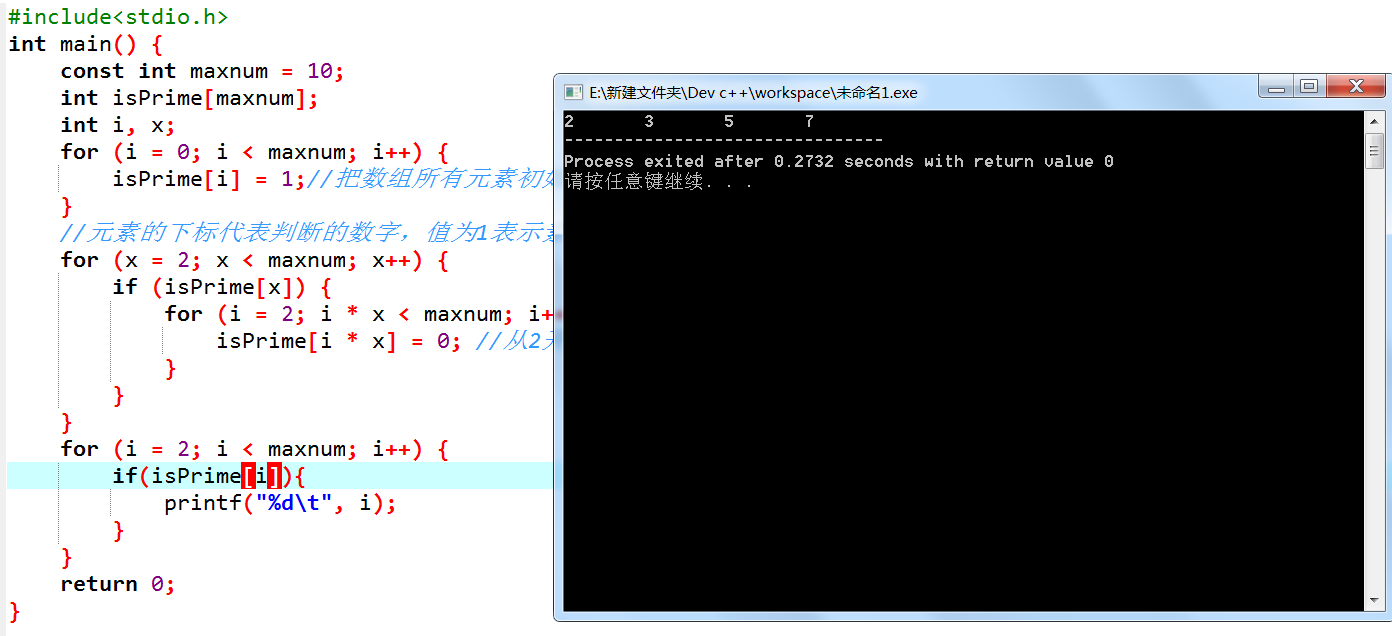2021-06-19 13:56

# C语言用数组的方法判断输入的十个数是不是素数

C语言用数组的方法判断输入的十个数是不是素数。。。。。。。。。。。。。。。

• 写回答
• 关注问题
• 收藏
• 邀请回答

#### 3条回答默认 最新

•CSDN专家-sinjack 2021-06-19 13:58
已采纳

如有帮助，请采纳。点击我回答右上角【采纳】按钮。

``````int main() {

const int maxnum = 10;
int isPrime[maxnum];
int i, x;
for (i = 0; i < maxnum; i++) {
isPrime[i] = 1;//把数组所有元素初始为1
}
//元素的下标代表判断的数字，值为1表示素数，值为0不是
for (x = 2; x < maxnum; x++) {
if (isPrime[x]) {
for (i = 2; i * x < maxnum; i++) {
isPrime[i * x] = 0; //从2开始，把每个数的倍数都挨个排除掉
}
}
}
for (i = 2; i < maxnum; i++) {
if(isPrime[i]){
printf("%d\t", i);
}
}
return 0;
}
``````已采纳该答案
打赏 评论
•``````bool isprime(int n)
{
for(int i=2;i<n;i++)
if(n%i==0)
return false;
return true;
}

void main()
{
int a,i;
bool prime = {0};
for(i=0;i<10;i++)
{
scanf("%d",&a[i]);
prime[i] = isprime(a[i]);
}
printf("输入的素数有：");
for(i=0;i<10;i++)
{
if(prime[i])
printf("%d ",a[i]);
}
}``````
打赏 评论
•有问必答小助手 2021-06-22 13:50

您好，我是有问必答小助手，您的问题已经有小伙伴解答了，您看下是否解决，可以追评进行沟通哦~

如果有您比较满意的答案 / 帮您提供解决思路的答案，可以点击【采纳】按钮，给回答的小伙伴一些鼓励哦～～